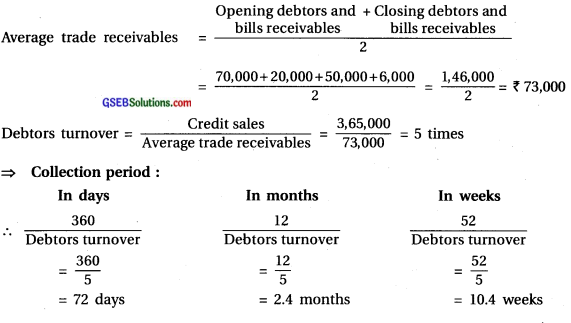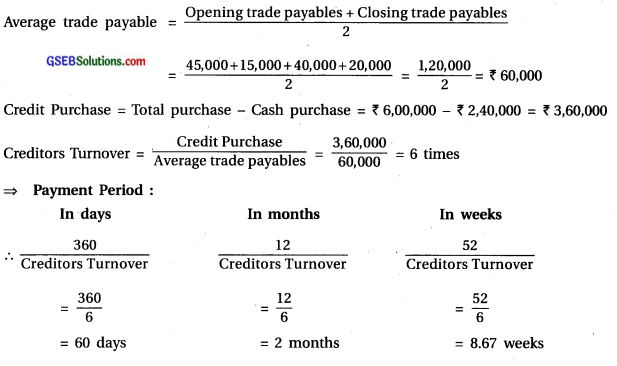# GSEB Solutions Class 12 Accounts Part 2 Chapter 5 Accounting Ratios and Analysis

Gujarat Board GSEB Textbook Solutions Class 12 Commerce Accounts Part 2 Chapter 5 Accounting Ratios and Analysis Textbook Exercise Questions and Answers.

## Gujarat Board Textbook Solutions Class 12 Accounts Part 2 Chapter 5 Accounting Ratios and Analysis

### GSEB Class 12 Accounts Accounting Ratios and Analysis Text Book Questions and Answers

1. Select the correct option for each question :

Question 1.
Which of the following is correct for accounting ratios?
(A) Comparison with ratios developed by the firm
(B) Comparison with ratios of industry
(C) Comparison with ratios of competitors
(D) All of the above
(D) All of the above

Question 2.
In which terms ratios are presented?
(A) Proportion
(B) Percentage
(C) Time
(D) All of the above
(D) All of the above

Question 3.
For which of the following items the ratio is computed in days?
(A) For total purchase
(B) For credit sales
(C) For credit purchase
(D) Both (B) and (C)
(D) Both (B) and (C)

Question 4.
Which of the following ratios are included in traditional classification?
(A) Composite ratios
(B) Liquidity ratios
(C) Profitability ratios
(D) Solvency ratios
(A) Composite ratiosQuestion 5.
Which of the following ratios is revenue-based profitability ratio?
(A) Gross profit ratio
(B) Net profit ratio
(C) Operating ratio
(D) Both (A) and (B)
(C) Operating ratio

Question 6.
A company has the purchase of ₹ 90,000, the purchase expenses of ₹ 15,000, the changes in stock (₹ 15,000) and sales of ₹ 1,50,000. Determine the gross profit ratio.
(A) 40%
(B) 13.33%
(C) 20%
(D) None of the above
(A) 40%

Question 7.
Which of the following is not included in operating expenses?
(A) Loss on sale of asset
(B) Loss due to fire
(C) Interest paid
(D) All of the above
(D) All of the above

Question 8.
The cost of goods sold of a company is ₹ 10,00,000. Operating expenses are ₹ 2,00,000. Non-operating expenses are ₹ 3,00,000. Financial expenses are ₹ 1,00,000. If total sales is ₹ 20,00,000, determine operating profit ratio.
(A) 20%
(B) 40%
(C) 30%
(D) 28%
(B) 40%

Question 9.
Liquidity ratio is ………………… .
(A) measurement of solvency
(B) measurement of short-term profitability
(C) measurement of profitability
(D) measurement of liquidity
(D) measurement of liquidity

Question 10.
Which of the following is not included to compute current ratio?
(A) Debtors
(B) Stock
(C) Bills receivables
(D) Furniture
(D) Furniture

Question 11.
Working capital means ………………… .
(A) difference between current assets and non-current assets
(B) difference between current liabilities and non-current assets
(C) difference between current assets and non-current liabilities
(D) difference between current assets and current liabilities.
(D) difference between current assets and current liabilities.Question 12.
To arrive at liquid assets which of the following is deducted from current assets?
(A) Stock
(B) Cash and cash equivalent
(C) Debtors
(D) Bills receivables
(A) Stock

2. Answer the following questions in one sentence :

Question 1.
What is ratio?
“The relationship between two accounting figures expressed mathematically is known as ratios.
– To make qualitative judgments about firms’ financial performance ratios is useful.

Question 2.
When ratio is useful?
Ratios are useful when they are compared with any standards, e.g. Ratios compared with ratios developed by the business entity, comparisons with ratios of industry and competitors etc.

Question 3.
In which forms ratios can be presented?
Ratios can be presented in the following ways.

• In terms of proportion
• In terms of percentage
• In terms of times
• In terms of fraction
• In terms of days/weeks/months.

Question 4.
What is cost of goods sold?
Cost of goods sold is determined as follows :

• Cost of goods sold = Sale – Gross profit OR sales + Gross loss
• Cost of goods sold = Wages + Factory expenses + Cost of goods consumed.

Question 5.
The gross profit ratio and the net profit ratios are revenue-based ratios or expense based ratios?
The gross profit ratio and the net profit ratios are revenue-based ratios.

Question 6.
When the changes in stock is positive whether it will be added to cost of goods sold or deducted?
When the changes in stock is positive then it will be added to cost of goods sold.

Question 7.
Give one illustration of financial expense.
Interest expense is an illustration of financial expense.

Question 8.
Are financial expenses included to determine operating ratio?
No, financial expenses are not included to determine operating ratio.

Question 9.
What is indicated by liquidity ratio?
Liquidity ratio shows the capacity of business unit to pay short term liabilities.

Question 10.
Will the current ratio increase or decrease when the current assets increase and the current liabilities remain unchanged?
Current ratio increases when the current assets increase and the current liabilities remain unchanged.

Question 11.
What is shareholder’s funds?
Shareholder’s funds is owner’s funds only which includes, equity shares capital, preference share capital reserves and surplus and deducted debit balance of P & L A/c.

Question 12.
What is indicated by interest coverage ratio?
Interest coverage ratio shows the relationship between profit before tax and interest on long term debts. This ratio also shows earning capacity.Question 13.
Which is better out of high-interest coverage ratio and low-interest coverage ratio?
High-interest coverage ratio is more better because it shows high payment capacity of interest of the business.

Question 14.
By which ratios efficiency is measured?
With the help of following ratios, efficiency is measured.

• Stock Turnover
• working capital turnover
• Debtors turnover
• Creditors turnover.

Question 15.
In order to arrive at trade, receivables is bad debt reserve deducted from debtors?
No, in order to arrive at trade receivable bad debts reserve will not be deducted from debtors.

3. Answer the following questions in brief :

Question 1.
Explain three objectives of ratio analysis.
Following are the objectives of ratio analysis.
1. To know profitability status of the entity: In accounting, the profitability of the business can be measured by applying the ratio analysis in different forms.

2. For financial statement analysis: Ratio analysis works as indicator to the users of financial statements. Different stakeholders undertake analysis of profit and loss statements and balance sheet. I make this analysis meaningful and useful ratio analysis is used.

3. To know the liquidity status of the entity: The liquidity ratio explains the capacity to meet short term liabilities of the entity. From the liquidity, short term solvency of the business can be measured.

4. To know operating efficiency of business entity: Different types of assets are acquired by the business entity, for measurement of effective and efficient use of assets, ratio analysis is useful. For this turnover ratio is used.

5. For forecasting: The trend of respective items can be seen by ratio analysis. This trend can be useful to the company to undertake planning and forecast.

6. The information about weak aspects: There may be overall good results of the business entity. Out of different aspects like profitability, liquidity, solvency or efficiency, there may be weak performance of anyone aspects and good performance of other aspects. By ratio analysis, each aspect is evaluated personally. So the information for weak aspects can be obtained and necessary remedies can be undertaken.

Question 2.
Describe three limitations of ratio analysis.
Main three limitations of ratio analysis can be as under :
1. Absence of standard ratios: Any individual ratio does not provide any analysis or interpretation. The use of ratios can be established only when the standard ratios are available for comparison. If standard ratios are not determined, the computed ratios become useless.

2. Influence of price changes: The base for ratio analysis is financial statement. Financial statements are prepared with historical information and in historical information the effect inflation is not considered which is also a limitation.

3. Difficulty in comparison: When two same category, business entities, follow different accounting policies, then information obtained by ratio analysis becomes useless, e.g. method of depreciation, method of stock valuation etc.

4. Limited Utility: The utility of ratios calculated on the basis of only one year is limited For the comparison the figures of the previous year and those of the current year is not much useful. If possible the figures of the firm ought to be compared with those of a firm similar business.

5. Factors Neglected: It is obvious that a business is affected by external and internal factors, competition and total economic condition of the country. But these factors are not included in the calculation of ratios. Since they are calculated on the past figures, they have limited utility.Question 3.
When are computed ratios useful?
Individual ratio does not indicate any type of favourable or unfavourable situation. For making analysis of financial statements more useful and meaningful comparison of ratio analysis is included in it. Individual ratio present only result but when computed ratio copmpared with any standards or with certified ratio then it is useful, i.e. current ratio of net profit is 25%, which gives only result, but in last year it was 20% and in current year it is 25% then we can compare it and get conclusion from it.

Question 4.
Explain the traditional classification of ratios.
In traditional classification, the computation of accounting ratio is done on the basis of financial statements. In this statement trading account, profit and loss account and balance sheet are included.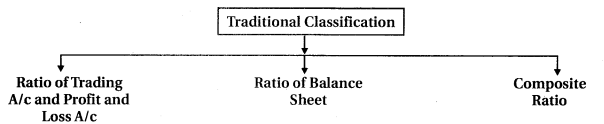• For computation of ratios of Trading A/c and profit-loss A/c values at numerator and denominator are taken from these accounts only. Similarly for balance sheet ratio, computation. values of numerator and denominator are taken from balance sheet only.
• For computation of composite ratios, one component is taken from Trading A/c or Profit and Loss A/c and other is taken from Balance Sheet.

Question 5.
Explain the functional classification of ratios.
Functional classification of ratios are as under :
1. Profitability Ratio: The earning capacity of a business unit is known as profitability. Following are the different ratios to measure profitability.

• Gross profit ratio
• Operating ratio
• Operating profit ratio
• Net profit ratio.

2. Liquidity Ratio: Liquidity means the ability of business unit to pay short-term liabilities or current liabilities. Following ratios are included in liquidity ratio :

• Current ratio
• Liquidity ratio.

(3) Solvency Ratio: The capacity of a business unit to pay the long-term liabilities is known as financial or economic solvency. Following ratio are included insolvency ratio :

• Debt to equity ratio
• Total assets to debt ratio
• Proprietary ratio
• Interest coverage ratio.

(4) Efficiency (Activity) Ratios: The profitability of the business increases, when all resources and equipment acquired by the business entity are used efficiently. Following ratios are included in efficiency ratio :

• Stock turnover
• Working capital turnover
• Debtor turnover
• Creditors turnover.

Question 6.
Explain any one ratio of profitability.
Profit earning capacity of the business is known as profitability. Profitability ratios are as under:

1. Gross profit ratio
2. Operating ratio
3. Operating profit ratio
4. Net profit ratio.
• Explanation of Gross profit ratio: Gross profit ratio shows the relationship between gross profit and total net sales. Gross profit ratio is an income-based ratio. Gross profit ratio indicates percentage of gross profit to sales. Sales means revenue from operation.
• Operating income means the income which is generated from the business in which company deals.
• Sales means net sales.

Formula : Gross profit ratio = $$\frac{\text { Gross profit }}{\text { Net Sales }} \times 100$$
Where, Gross profit = Total Sales – Cost of goods sold
Total sales = Credit sales + Cash sales
Net sales = Sales – Sales return
Cost of goods sold = Opening stock of raw material + purchase of raw material + purchase expenses + wages+ factory expenses – closing stock of raw materialQuestion 7.
Explain any one ratio of liquidity.
Liquidity means the ability of business unit to pay short term liabilities. Short term liabilities means current liabilities. Liquidity ratios are
1. Current ratio
2. Liquid ratio.

Explanation of Current Ratio: Current ratio shows the relationship between current assets and current liabilities. If the proportion of current assets is higher than current liabilities then the liquidity position of business entity is good and more liquidity means more short-terms solvency.

Formula : Current ratio = $$\frac{\text { Currrent Assets }}{\text { Current Liabilities }}$$
Current assets means that which is in the cash form or cash equivalent or which can be converted into cash within 12 months or which can be converted into cash equivalent. Examples of Current Assets: Current investment, stock (Excluding loose tools), trade receivables, cash and cash equivalent, short term lendings and advances, expenses paid in advance, incomes outstanding, taxes paid in advance etc.
Current liabilities means such liabilities which are payable within the time period of 12 months.

• Examples of Current Liabilities: Short term borrowings, trade payables, interest payable on long term liabilities, due but not paid liability, due but not paid interest, expenses outstandings, uncalled dividend, Installments received in advance, short-term provisions etc.
• Proportions for liquidity ratio 2: 1 is desirable
• The difference of current assets and current liabilities is known as working capital.

Question 8.
Explain any one ratio of solvency.
Solvency ratio is used to measure how far the business entity is competent enough to pay long term liabilities. Following are the solvency ratios.

• Debt to equity ratio,
• Total assets to debt ratio,
• Proprietary ratio,
• Interest coverage ratio.

Explanation of Debt-equity ratio :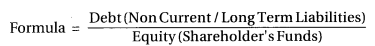• The objective of this ratio is to know the proportion of owners capital and borrowed funds which are acquired by the business as a long term source of finance.
• Here long term liabilities means – long term borrowings deferred tax liabilities, other long term liability and long term provisions etc.
• Shareholders funds = Equity share Capital + Preference share capital + Reserves and surplus
• Debit balance of profit-loss A/c
• If debt to equity ratio is higher, it indicates that the business unit is more dependent on the borrowed- funds and it is risky from the lenders point of view.
• If debt to equity ratio is low, it means business unit is less dependent on the borrowed funds and it is not risky from the lenders’ point of view.

Question 9.
Explain any one ratio of efficiency.
The profitability of the business increases, when all resources and equipments acquired by the business entity are used efficiently. In this context efficiency or activity ratios are developed. Examples of efficiency ratios are as follows :

• Stock turnover
• Working capital turnover
• Debtors turnover
• Creditors turnover.

Explanation of Stock turnover :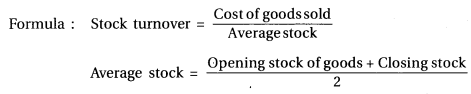• Cost of goods sold (COGs) = Sales – Gross profit
= Sales + gross loss (If it is given)
• COGS = Opening stock of goods + purchase of raw material + purchase expense + wages + factory expenses – closing stock of raw material
• This ratio is the measurement of efficiency for the conversion of stock into sales.
• For this ratio, it is established that higher the ratio – higher the sales – higher the profit.Question 10.
What are included in operating expenses?
Operating expenses are those expenses which are incurred for operating activities of business.
– Operating expenses includes- office/administrative expenses, Sales distribution expenses, Employees benefit expenses, depreciation, amount written off etc.

Question 11.
Explain non-operating incomes.
Income or revenue arise from activity except business activity of an unit is know as non¬operating incomes. Non operating incomes are like interest received, dividend received, profit on sales of asset should not be considered for the determination of operating cost.

Question 12.
Do we consider current investments for current ratio?
Yes, we have to consider current investment for current ratio. Because in calculation of current ratio we consider current assets and current investments are included in current assets.

Question 13.
Explain the approaches of proprietary funds.
There are two approaches used to determine the owner’s funds. Under both the approaches owners’ funds remain identical.
1. Liabilities Based Approach: As per this approach ;
Owners’ funds = Equity share capital + Preference share capital + Reserves and surplus – Debt balance of P & L A/c (if any).

2. Assets Based Approach: As per this approach ;
Owners’ funds = Non-current Assets + Current Assets – Non-current liabilities – Current liabilities.

Question 4.
Determine gross profit ratio :
(1) Sales ₹ 10,00,000,
(2) Sales ₹ 15,00,000,
(3) Sales ₹ 20,00,000,
Operating stock ₹ 2,50,000,
Purchase ₹ 12,00,000,
Gross profit ₹ 3,00,000
Cost of goods sold ₹ 12,00,000
Sales return ₹ 2,00,000
Closing stock ₹ 3,50,000
Purchase expenses ₹ 50,000
1. Gross Profit Ratio = $$\frac{\text { Gross Profit }}{\text { Net Sales }} \times 100=\frac{3,00,000}{10,00,000} \times 100=30 \%$$

2. Gross Profit = Sales – Cost of goods sold = ₹ 15,00,000 – ₹ 12,00,000 = ₹ 3,00,000
∴ Gross Profit Ratio = $$\frac{\text { Gross Profit }}{\text { Net Sales }} \times 100=\frac{3,00,000}{15,00,000} \times 100=20 \%$$

3. Cost of goods sold = Opening stock + Purchase + Purchase expenses – Closing stock = ₹ 2,50,000 + ₹ 12,00,000 + ₹ 50,000 – ₹ 3,50,000 = ₹ 11,50,000
Net Sales = Sales – Sales return = ₹ 20,00,000 – ₹ 2,00,000 = ₹ 18,00,000 Gross Profit = Sales – Cost of goods sold = ₹ 18,00,000 – ₹ 11,50,000 = ₹ 6,50,000

Question 5.
From the following information calculate operating ratio :

 Particulars (₹) Particulars (₹) Non-operating other expenses 35,000 Income of interest-dividend 45,000 Depreciation 48,000 Sales expenses 52,000 Administrative expenses 75,000 Cost of goods sold 3,25,000 Sales 6,00,000 Financial expenses 85,000 Tax rate 30%

Operating expense = Administrative expense + Sales expense + Depreciation = ₹ 75,000 + ₹ 52,000 + ₹ 48,000 = ₹ 1,75,000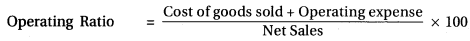= $$\frac{3,25,000+1,75,000}{6,00,000} \times 100=\frac{5,00,000}{6,00,000} \times 100$$ = 83.33%.Question 6.
From the following information calculate (i) operating ratio and (ii) operating profit ratio:

 Particulars (₹) Particulars (₹) Sales 39,00,000 Wages 2,00,000 Cost of goods consumed 25,00,000 Administrative expenses 1,75000 Sales expenses 75,000 Interest on loan 60,000 Loss due to accident 40,000 Dividend received on investments 1,00,000 Factory expenses including Tax rate 30% depreciation 50,000

Cost of goods sold = Cost of goods consumed + Wages
= ₹ 25,00,000 + ₹ 2,00,000 = ₹ 27,00,000
Operating Expense = Administrative expense + Sales expense + Factory expenses (including
depreciation)
= ₹ 1,75,000 + ₹ 75,000 + ₹ 50,000 = ₹ 3,00,000
(i) Operating Ratio = img
= $$\frac{27,00,000+3,00,000}{39,00,000} \times 100$$
= $$\frac{30,00,000}{39,00,000}$$ = 76.92%
Operating cost = Cost of goods sold + Operating expenses = ₹ 27,00,000 + ₹ 3,00,000 = ₹ 30,00,000
Operating Profit= Sales – Operating Cost = ₹ 39,00,000 – ₹ 30,00,000 = ₹ 9,00,000
Operating Profit Ratio = $$\frac{\text { Operating Profit }}{\text { Net Sales }} \times 100$$
= $$\frac{9,00,000}{39,00,000} \times 100$$ = 23.8% OR
Operating Profit Ratio = 100% – Operating Ratio
= 100 – 76.92 = 23.08%

Question 7.
Calculate net profit ratio from information of questions no. 5 and 6.
To find out profit after tax (based on Que. 5)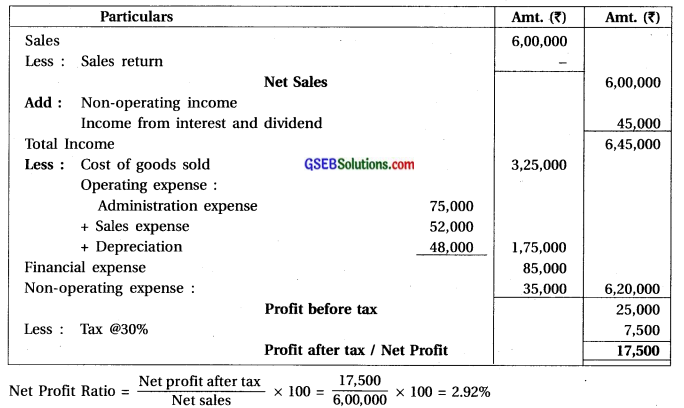To find out profit after tax (based on Que. 6)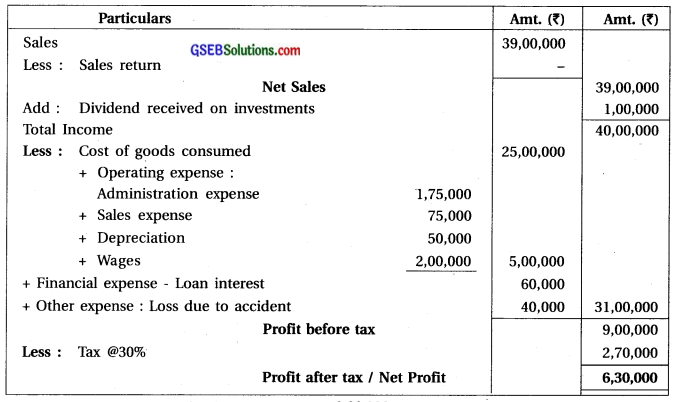Net Profit Ratio = $$\frac{\text { Net profit after tax }}{\text { Net sales }} \times 100=\frac{6,30,000}{39,00,000} \times 100$$ = 16.15%

Question 8.
From the following information calculate current ratio and liquid ratio:

 Particulars (₹) Particulars (₹) Stock 3,00,000 Bills receivables 75,000 Debtors 2,50,000 Bad debts reserve 20,000 Cash and cash equivalent 1,20,000 Expenses paid in advance 60,000 Furniture 1,60,000 CredItors 2,00,000 Bills payable 60,000 Outstanding expenses, 50,000 Short-term loan 40,000

Current ratio = $$\frac{\text { Current assets }}{\text { Current liabilities }}$$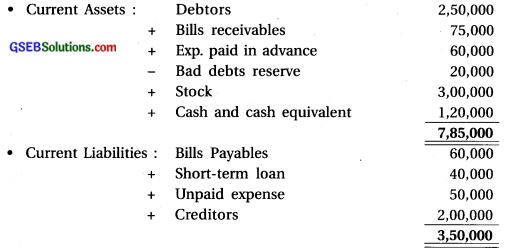Current Ratio = $$\frac{\text { Current assets }}{\text { Current liabilities }}=\frac{7,85,000}{3,50,000}$$ = 2.24

Liquid Ratio:
Liquid Assets = Current Assets ₹ 7,85,000 – ₹ 3,60,000 (stock ₹ 3,00,000 + Exp.paid in Advance ₹ 60,000 ) = ₹ 4,25,000

Liquid Ratio = $$\frac{\text { Liquid Assets }}{\text { Liquid Liabilities }}=\frac{4,25,000}{3,50,000}$$ = 1.21:1Question 9.
From the following information of ‘H’ Limited, calculate debt-equity ratio :

 Particulars (₹) Particulars (₹) Long-term liabilities 8,00,000 Equity share capital 8,00,000 Long-term provisions 4,00,000 Preference share capital 2,00,000 Debit balance of profit-loss A/c 50,000 Creditors 1,25,000 Bills payables 25,000 Outstanding expenses 10,000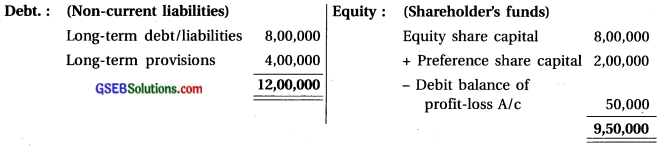Debt-equity ratio = $$\frac{\text { Debt (Non-current liabilities) }}{\text { Equity (Shareholder’s funds) }}=\frac{12,00,000}{9,50,000}$$ = 126 : 1

Question 10.
From the following information of ‘M’ Limited, calculate debt-equity ratio :

 Particulars (₹) Particulars (₹) Current liabilities 4,50,000 Long-term liabilities 8,00,000 Long-term provisions 6,00,000 Total assets 30,00,000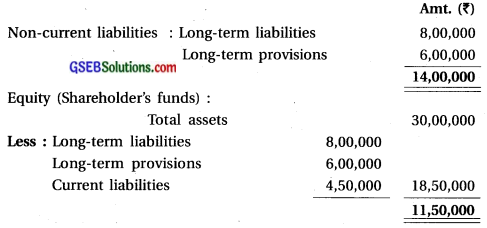Debt equity ratio = $$\frac{\text { Debt (Non-current liabilities) }}{\text { Equity (Shareholder’s funds) }}=\frac{14,00,000}{11,50,000}$$ = 1.22:1

Question 11.
From the following information of’N Company Limited, calculate debt-equity ratio:

 Particulars (₹) Particulars (₹) Creditors 1,60,000 Non-current assets 12,00,000 Bills payables 1,40,000 Current assets 10,00,000 Outstanding expenses 1,00,000 Total liabilities 10,00,000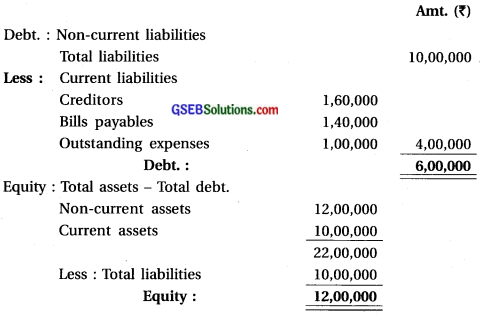Debt equity ratio = $$\frac{\text { Debt (Non-current liabilities) }}{\text { Equity (Shareholder’s funds) }}=\frac{6,00,000}{12,00,000}$$ = 0.5:1

Question 12.
From the following information of ‘R’ Company Limited, calculate total assets-debt ratio :

 Particulars (₹) Particulars (₹) Preference Share Capital 10,00,000 Non-current liabilities 4,00,000 Equity Share Capital 6,00,000 Current liabilities 4,00,000 Reserve and surplus 2,00,000

In the sum total assets value is not given but detail of capital liabilities side are given. Therefore, total of capital-liabilities side will be considered as total of assets.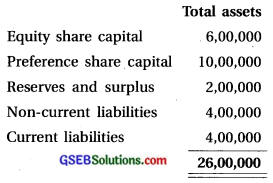Total assets – debt ratio = $$\frac{\text { Total assets }}{\text { Debt (Long term liabilities / Non-current liabilities) }}=\frac{26,00,000}{4,00,000}$$ = 6.5:1Question 13.
From the following information of‘T’ Company Limited, calculate proprietary ratio :

 Particulars (₹) Particulars (₹) Equity share capital 12,00,000 Current liabilities 4,00,000 Preference share capital 8,00,000 Non-current assets 12,00,000 Reserve and surplus 4,00,000 Current assets 24,00,000 Non-current liabilities 8,00,000

Owner’s funds (Shareholder’s funds) = Equity share capital ₹ 12,00,000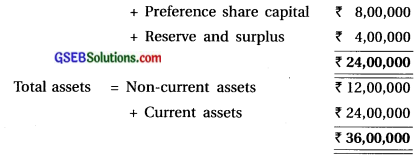Proprietary ratio = $$\frac{\text { Owner’s funds }}{\text { Total assets }}=\frac{24,00,000}{36,00,000}$$ = 36,00,000 = 0.66 : 1

Question 14.
From the following information of ‘K’ Company Limited, calculate interest coverage ratio :

 Particulars (₹) Particulars (₹) Profit after tax 7,50,000 Taxes paid 2,50,000 10% Debentures 20,00,000 12% Loan 10,00,000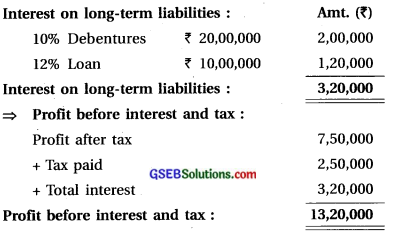Interset coverage ratio = $$\frac{\text { Profit before interest and tax }}{\text { Interest on long-term liabilities }}=\frac{13,20,000}{3,20,000}$$ = 4.125 times

Question 15.
Calculate stock turnover ratio from the following information of ‘L’ Company Limited :

 Particulars (₹) Particulars (₹) Sales 30,00,000 Opening stock 3,50,000 Closing stock 2,50,000 Purchases 12,00,000 Gross profit rate 30%

Cost of goods sold = Sales – Gross Profit (@ 30%) = ₹ 30,00,000 – ₹ 9,00,000 = ₹ 21,00,000
Average Stock = $$\frac{\text { Opening stock + Closing stock }}{2}$$ = $$\frac{3,50,000+2,50,000}{2}$$ = ₹ 3,00,000
Stock Turnover = $$\frac{\text { Cost of goods sold }}{\text { Average stock }}=\frac{21,00,000}{3,00,000}$$ = 7 times

Question 16.
Calculate stock turnover ratio from the following information of ‘Y’ Company Limited :

 Particulars (₹) Particulars (₹) Opening stock 2,00,000 Closing stock 1,50,000 Sales 40,00,000 Purchases 22,00,000 Purchase expenses 1,00,000 Wages 2,50,000

Average stock = $$\frac{\text { Opening stock }+\text { Closing stock }}{2}=\frac{2,00,000+1,50,000}{2}$$ = ₹ 1,75,000
Cost of goods sold = Opening stock + Purchase + Purchase expense + Wages – Closing stock = ₹ 2,00,000 + ₹ 22,00,000 + ₹ 1,00,000 + ₹ 2,50,000 – ₹ 1,50,000 = ₹ 26,00,000
Stock turnover ratio = $$\frac{\text { Cost of goods sold }}{\text { Average stock }}=\frac{26,00,000}{1,75,000}$$ = 14.86 times

Question 17.
Calculate working capital turnover ratio (based In sales and cost of goods sold), from the following information of ‘Z’ Company Limited:

 Particulars (₹) Particulars (₹) Cost of goods sold 32,00,000 Gross profit 8,00,000 Non-current assets 10,00,000 Current assets 5,00,000 Non-current liabilities 6,00,000 Current liabilities 3,00,000

Working capital turnover (based on sales) :
Cost of goods sold = Sales – Gross profit
∴ Sales = Cost of goods sold + Gross profit = ₹ 32,00,000 + ₹ 8,00,000 = ₹ 40,00,000
Working capital = Current assets – Current liabilities = ₹ 5,00,000 – ₹ 3,00,000 = ₹ 2,00,000
∴ Working capital turnover =$$\frac{\text { Sales }}{\text { Working capital }}=\frac{40,00,000}{2,00,000}$$ = 20 times
⇒ Working capital turnover (based on cost of goods sold) :
Working capital = Current assets – Current liabilities = ₹ 5,00,000 – ₹ 3,00,000 = ₹ 2,00,000
Cost of goods sold = ₹ 32,00,000
∴ Working caPital turnover = $$\frac{\text { Sales (Cost of goods sold) }}{\text { Working capital }}=\frac{32,00,000}{2,00,000}$$ = 16 times.

Question 18.
From the following information of ‘B’ Company Limited, calculate debtors turnover and collection period in days. What will be answer if collection period is calculated in terms of weeks and months? (Assume 360 days of the year)

 Particulars (₹) Particulars (₹) Total Sales 4,50,000 Credit Sales 3,65,000 Opening debtors 70,000 Closing debtors 50,000 Opening bills receivables 20,000 Closing bills receivables 6,000 pening creditors 45,000 Closing creditors 48,000